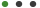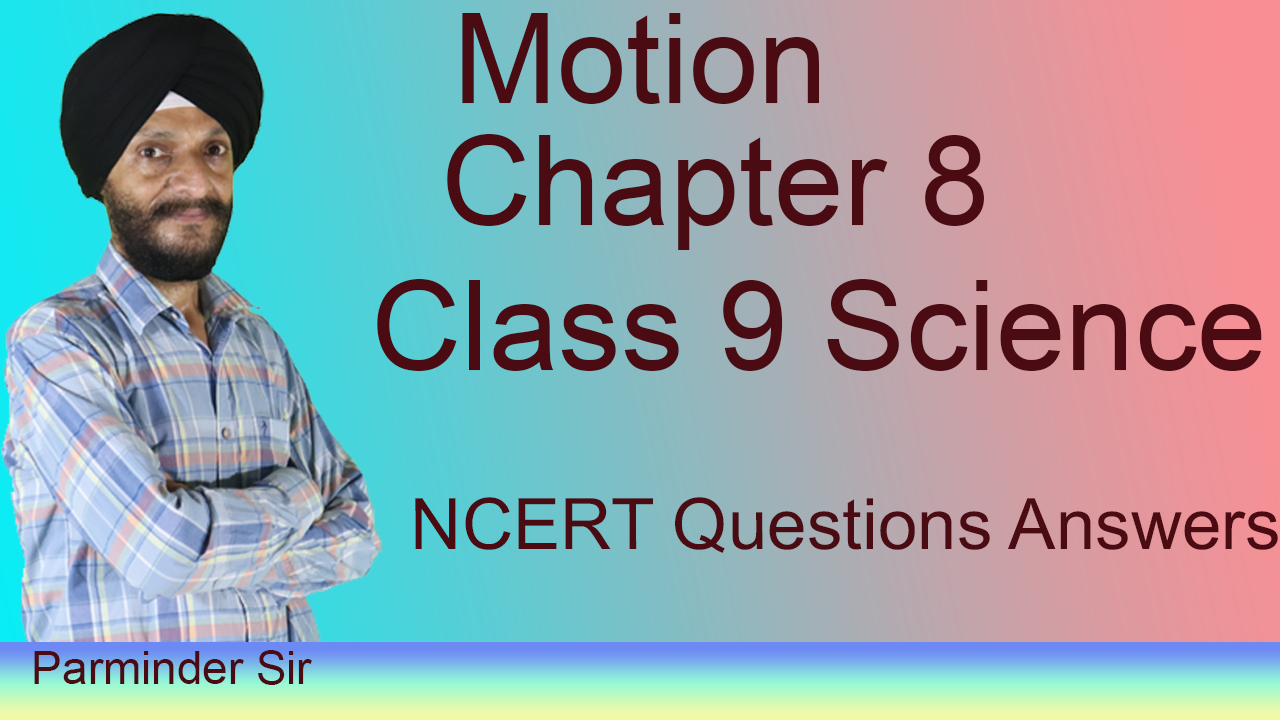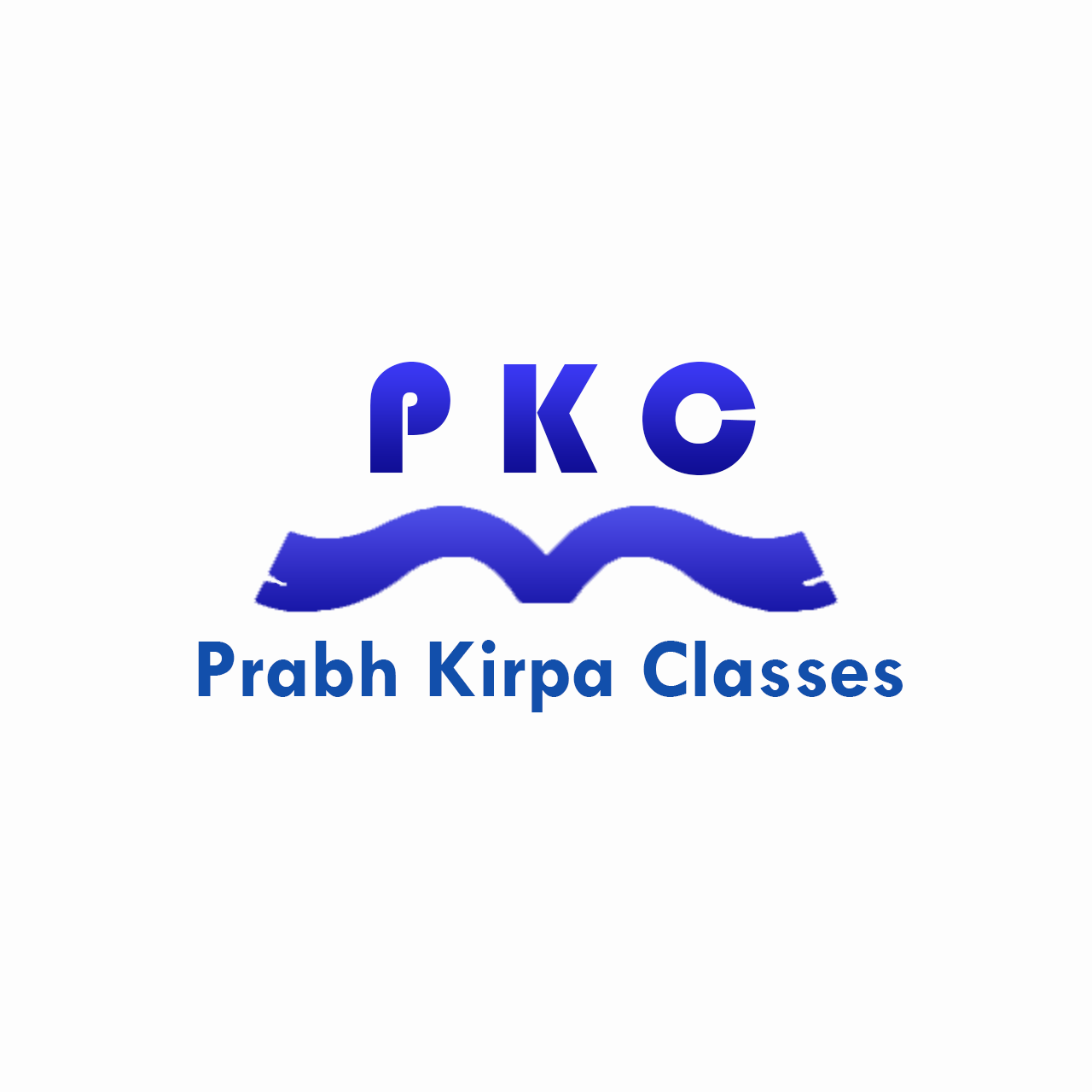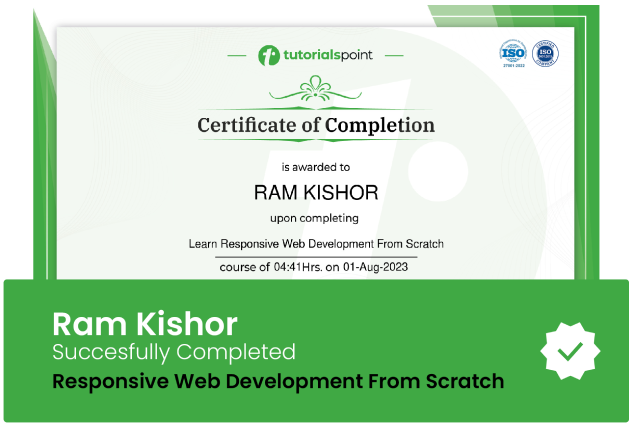### Motion Class 9 Science NCERTPrabh Kirpa Classes

# Motion Class 9 Science NCERT

Motion Class 9 Science NCERTUpdated on Sep, 2023Language - EnglishPrabh Kirpa ClassesTeaching & Academics,Physics,ScienceTraining 5 or more people ?

## Course Description

In everyday life, we see some objects at rest and others in motion. Birds fly, fish swim, blood flows through veins and arteries, and cars move. Atoms, molecules, planets, stars, and galaxies are all in motion. We often perceive an object to be in motion when its position changes with time. However, there are situations where the motion is inferred through indirect evidences. For example, we infer the motion of air by observing the movement of dust and the movement of leaves and branches of trees. What causes the phenomena of sunrise, sunset, and changing of seasons? Is it due to the motion of the earth? If it is true, why don’t we directly perceive the motion of the earth? An object may appear to be moving for one person and stationary for some other. For the passengers in a moving bus, the roadside trees appear to be moving backward. A person standing on the roadside perceives the bus along with the passengers as moving. However, a passenger inside the bus sees his fellow passengers be at rest. What do these observations indicate? Most motions are complex. Some objects may move in a straight line, others may take a circular path. Some may rotate and a few others may vibrate. There may be situations involving a combination of these. In this chapter, we shall first learn to describe the motion of objects along a straight line. We shall also learn to express such motions through simple equations and graphs. Later, we shall discuss ways of describing evidence.

We describe the location of an object by specifying a reference point. Let us understand this by an example. Let us assume that a school in a village is 2 km north of the railway station. We have specified the position of the school with respect to the railway station. In this example, the railway station is the reference point. We could have also chosen other reference points according to our convenience. Therefore, to describe the position of an object we need to specify a reference point called the origin

The rate of motion of an object can be more comprehensive if we specify its direction of motion along with its speed. The quantity that specifies both these aspects is called velocity. Velocity is the speed of an object moving in a definite direction. The velocity of an object can be uniform or variable. It can be changed by changing the object’s speed, direction of motion or both. When an object is moving along a straight line at a variable speed, we can express the magnitude of its rate of motion in terms of average velocity. It is calculated in the same way as we calculate average speed. In case the velocity of the object is changing at a uniform rate, then average velocity is given by the arithmetic mean of initial velocity and final velocity for a given period of time.

### Goals

What will you learn in this course:

• What is the difference between distance and displacement?
• What is the difference between speed and velocity?
• What are the three equations of Motion?
• How we can calculate distance, displacement, speed, velocity, acceleration, time in different situations?
• What are show uniform and non-uniform acceleration?
• What is the quantity which is measured by the area occupied below the velocity-time graph?
• are show What do the distance-time graph and speed-time graphs the ? etc. + many numerical type questions relating to the motion of topic.

### Prerequisites

What are the prerequisites for this course?

• No Requirements## Curriculum

Check out the detailed breakdown of what’s inside the course

Introduction
1 Lectures
•Introduction 01:32 01:32
Distance, Displacement, velocity, speed, acceleration, perimeter
1 LecturesNumericals of distance time , v-t , speed time graph, three equations of motion
1 LecturesFinding distance & displacement, average speed and velocity, velocity time graph
1 LecturesDistance time graph, speed time graph etc.
1 Lectures## Instructor DetailsPrabh Kirpa Classes

This channel is purely education based. Our aim is to ensure that the students are able to understand each and every question in an easy way.  We take pride in providing quality educational services to the students of Class VII to Class XI  Science NCERT, English, Computer Solutions, Mathematics & Business Studies.  Our goal is to help the leaders of tomorrow to learn to succeed today.

## Course Certificate

User your certification to make a career change or to advance in your current career. Salaries are among the highest in the world.